Advertisement Remove all ads

# HC Verma solutions for Class 11, 12 Concepts of Physics 1 chapter 17 - Light Waves [Latest edition]

Advertisement Remove all ads

#### Chapters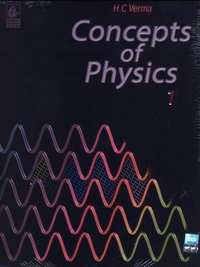## Chapter 17: Light Waves

Short AnswersMCQOthers
Short Answers [Page 379]

### HC Verma solutions for Class 11, 12 Concepts of Physics 1 Chapter 17 Light Waves Short Answers [Page 379]

Short Answers | Q 1 | Page 379

Is the colour of 620 nm light and 780 nm light same? Is the colour of 620 nm light and 621 nm light same? How many colours are there in white light?

Short Answers | Q 2 | Page 379

The wavelength of light in a medium is $\lambda = \lambda_0 /\mu,$ where $\lambda$ is the wavelength in vacuum. A beam of red light $\left( \lambda_0 = 720\text{ nm} \right)$ enters water. The wavelength in water is $\lambda = \lambda_0 /\mu = 540\text{ nm.}$ To a person under water, does this light appear green?

Short Answers | Q 3 | Page 379

Will the diffraction effects from a slit be more or less clearly visible if the slit-width is increased?

Short Answers | Q 4 | Page 379

If we put a cardboard (say 20 cm × 20 cm) between a light source and our eyes, we can't see the light. But when we put the same cardboard between a sound source and out ear, we hear the sound almost clearly. Explain.

Short Answers | Q 5 | Page 379

TV signals broadcast by a Delhi studio cannot be directly received at Patna, which is about 1000 km away. But the same signal goes some 36000 km away to a satellite, gets reflected and is then received at Patna. Explain.

Short Answers | Q 6 | Page 379

Can we perform Young's double slit experiment with sound waves? To get a reasonable "fringe pattern", what should be the order of separation between the slits? How can the bright fringes and the dark fringes be detected in this case?

Short Answers | Q 7 | Page 379

Is it necessary to have two waves of equal intensity to study interference pattern? Will there be an effect on clarity if the waves have unequal intensity?

Short Answers | Q 8 | Page 379

Can we conclude from the interference phenomenon whether light is a transverse wave or a longitudinal wave?

Short Answers | Q 9 | Page 379

Why don't we have interference when two candles are placed close to each other and the intensity is seen on a distant screen? What happens if the candles are replaced by laser sources?

Short Answers | Q 10 | Page 379

If the separation between the slits in a Young's double slit experiment is increased, what happens to the fringe-width? If the separation is increased too much, will the fringe pattern remain detectable?

Short Answers | Q 11 | Page 379

Suppose white light falls on a double slit but one slit is covered by a violet filter (allowing λ = 400 nm). Describe the nature of the fringe pattern observed.

Advertisement Remove all ads
MCQ [Pages 379 - 380]

### HC Verma solutions for Class 11, 12 Concepts of Physics 1 Chapter 17 Light Waves MCQ [Pages 379 - 380]

MCQ | Q 1 | Page 379

Light is _______________ .

• a wave phenomenon

• a particle phenomenon

• both a particle and a wave phenomenon

MCQ | Q 2 | Page 379

The speed of light depends ____________ .

• on elasticity of the medium only

• on inertia of the medium only

• on elasticity as well as inertia

• neither on elasticity nor on inertia

MCQ | Q 3 | Page 379

The equation of a light wave is written as $y = A \sin\left( kx - \omega t \right).$ Here, y represents _______ .

• displacement of ether particles

• pressure in the medium

• density of the medium

• electric field

MCQ | Q 4 | Page 379

Which of the following properties shows that light is a transverse wave?

• Reflection

• Interference

• Diffraction

• Polarization

MCQ | Q 5 | Page 379

When light is refracted into a medium, ______________ .

• its wavelength and frequency increase

• its wavelength increases but frequency remains unchanged

• its wavelength decreases but frequency remains unchanged

• its wavelength and frequency decrease

MCQ | Q 6 | Page 379

When light is refracted, which of the following does not change?

• Wavelength

• Frequency

• Velocity

• Amplitude

MCQ | Q 7 | Page 379

An amplitude modulated (AM) radio wave bends appreciably round the corners of a 1 m × 1 m board but a frequency modulated (FM) wave only bends negligibly. If the average wavelengths of the AM and FM waves are $\lambda_a and \lambda_f,$

• $\lambda_a > \lambda_f$

• $\lambda_a = \lambda_f$

• $\lambda_a < \lambda_f$

• We don't have sufficient information to evaluate the relation of $\lambda_a\text{ and }\lambda_f.$

MCQ | Q 8 | Page 379

Which of the following sources provides the best monochromatic light?

• A candle

• A bulb

• A mercury tube

• A laser

MCQ | Q 9 | Page 379

The wavefronts of a light wave travelling in vacuum are given by x + y + z = c. The angle made by the direction of propagation of light with the X-axis is _________ .

• 45°

• 90°

• $\cos^{- 1} \left( 1/\sqrt{3} \right)$

MCQ | Q 10 | Page 379

The wavefronts of light coming from a distant source of unknown shape are nearly _________ .

• plane

• elliptical

• cylindrical

• spherical

MCQ | Q 11 | Page 379

The inverse square law of intensity $\left(\text{i.e. the intensity }\infty \frac{1}{r^2}\right)$ is valid for a ____________ .

• point source

• line source

• plane source

• cylindrical source

MCQ | Q 12 | Page 379

Two sources are called coherent if they produce waves ______________ .

• of equal wavelength

• of equal velocity

• having same shape of wave front

• having a constant phase difference

MCQ | Q 13 | Page 379

When a drop of oil is spread on a water surface, it displays beautiful colours in daylight because of ______________ .

• disperson of light

• reflection of light

• polarization of light

• interference of light

MCQ | Q 14 | Page 380

Two coherent sources of different intensities send waves which interfere. The ratio of maximum intensity to the minimum intensity is 25. The intensities of the sources are in the ratio ____________ .

• 25 : 1

• 5 : 1

• 9 : 4

• 625 : 1

MCQ | Q 15 | Page 380

The slits in a Young's double slit experiment have equal width and the source is placed symmetrically with respect to the slits. The intensity at the central fringe is I0. If one of the slits is closed, the intensity at this point will be ____________ .

• I_0

• I_0/4

• I_0/2

• 4I_0

MCQ | Q 16 | Page 380

A thin transparent sheet is placed in front of a Young's double slit. The fringe-width will _____________ .

• increase

• decrease

• remain same

• become non-uniform

MCQ | Q 17 | Page 380

If Young's double slit experiment is performed in water, _________________ .

• the fringe width will decrease

• the fringe width will increase

• the fringe width will remain unchanged

• there will be no fringe

Advertisement Remove all ads
MCQ [Page 380]

### HC Verma solutions for Class 11, 12 Concepts of Physics 1 Chapter 17 Light Waves MCQ [Page 380]

MCQ | Q 1 | Page 380

A light wave can travel

(a) in vacuum

(b) in vacuum only

(c) in a material medium

(d) in a material medium only

MCQ | Q 2 | Page 380

Which of the following properties of light conclusively support the wave theory of light?

(a) Light obeys the laws of reflection.

(b) Speed of light in water is smaller than its speed in vacuum.

(c) Light shows interference.

(d) Light shows photoelectric effect.

MCQ | Q 3 | Page 380

When light propagates in vacuum, there is an electric field as well as a magnetic field. These fields ____________ .

(a) are constant in time

(b) have zero average value

(c) are perpendicular to the direction of propagation of light.

(d) are mutually perpendicular

MCQ | Q 4 | Page 380

Huygens' principle of secondary wavelets may be used to

(a) find the velocity of light in vacuum

(b) explain the particle behaviour of light

(c) find the new position of a wavefront

(d) explain Snell's Law

MCQ | Q 5 | Page 380

Three observers A, B and C measure the speed of light coming from a source to be νA, νBand νC. A moves towards the source and C moves away from the source at the same speed. B remains stationary. The surrounding space is vacuum everywhere.

(a) $\nu_A > \nu_B > \nu_C$

(b) $\nu_A < \nu_B < \nu_C$

(c) $\nu_A = \nu_B = \nu_C$

(d) $\nu_B = \frac{1}{2}\left( \nu_A + \nu_C \right)$

MCQ | Q 6 | Page 380

Three observers A, B and C measure the speed of light coming from a source to be νA, νBand νC. A moves towards the source and C moves away from the source at the same speed. B remains stationary. The surrounding space is water everywhere.

(a) $\nu_A > \nu_B > \nu_C$

(b) $\nu_A < \nu_B < \nu_C$

(c) $\nu_A = \nu_B = \nu_C$

(d) $\nu_B = \frac{1}{2}\left( \nu_A + \nu_C \right)$

MCQ | Q 7 | Page 380

Light waves travel in vacuum along the X-axis. Which of the following may represent the wave fronts?

• x = c

• y = c

• z = c

• x + y + z = c

MCQ | Q 8 | Page 380

If the source of light used in a Young's double slit experiment is changed from red to violet, ___________ .

• the fringes will become brighter

• consecutive fringes will come closer

• the intensity of minima will increase

• the central bright fringe will become a dark fringe

MCQ | Q 9 | Page 380

A Young's double slit experiment is performed with white light.

(a) The central fringe will be white.

(b) There will not be a completely dark fringe.

(c) The fringe next to the central will be red.

(d) The fringe next to the central will be violet.

MCQ | Q 10 | Page 380

Four light waves are represented by

(i) $y = a_1 \sin \omega t$

(ii) $y = a_2 \sin \left( \omega t + \epsilon \right)$

(iii) $y = a_1 \sin 2\omega t$

(iv) $y = a_2 \sin 2\left( \omega t + \epsilon \right).$

Interference fringes may be observed due to superposition of

(a) (i) and (ii)

(b) (i) and (iii)

(c) (ii) and (iv)

(d) (iii) and (iv)

Advertisement Remove all ads
[Pages 380 - 383]

### HC Verma solutions for Class 11, 12 Concepts of Physics 1 Chapter 17 Light Waves [Pages 380 - 383]

Q 1 | Page 380

Find the range of frequency of light that is visible to an average human being

$\left( 400\text{ nm }< \lambda < 700\text{ nm}\right)$

Q 2 | Page 380

The wavelength of sodium light in air is 589 nm. (a) Find its frequency in air. (b) Find its wavelength in water (refractive index = 1.33). (c) Find its frequency in water. (d) Find its speed in water.

Q 3 | Page 380

The index of refraction of fused quartz is 1.472 for light of wavelength 400 nm and is 1.452 for light of wavelength 760 nm. Find the speeds of light of these wavelengths in fused quartz.

Q 4 | Page 380

The speed of yellow light in a certain liquid is 2.4 × 108 m s−1. Find the refractive index of the liquid.

Q 5 | Page 380

Two narrow slits emitting light in phase are separated by a distance of 1⋅0 cm. The wavelength of the light is $5 \cdot 0 \times {10}^{- 7} m.$ The interference pattern is observed on a screen placed at a distance of 1.0 m. (a) Find the separation between consecutive maxima. Can you expect to distinguish between these maxima? (b) Find the separation between the sources which will give a separation of 1.0 mm between consecutive maxima.

Q 6 | Page 381

The separation between the consecutive dark fringes in a Young's double slit experiment is 1.0 mm. The screen is placed at a distance of 2.5m from the slits and the separation between the slits is 1.0 mm. Calculate the wavelength of light used for the experiment.

Q 7 | Page 381

In a double slit interference experiment, the separation between the slits is 1.0 mm, the wavelength of light used is 5.0 × 10−7 m and the distance of the screen from the slits is 1.0m. (a) Find the distance of the centre of the first minimum from the centre of the central maximum. (b) How many bright fringes are formed in one centimetre width on the screen?

Q 8 | Page 381

In a Young's double slit experiment, two narrow vertical slits placed 0.800 mm apart are illuminated by the same source of yellow light of wavelength 589 nm. How far are the adjacent bright bands in the interference pattern observed on a screen 2.00 m away?

Q 9 | Page 381

Find the angular separation between the consecutive bright fringes in a Young's double slit experiment with blue-green light of wavelength 500 nm. The separation between the slits is $2 \cdot 0 \times {10}^{- 3}m.$

Q 10 | Page 381

A source emitting light of wavelengths 480 nm and 600 nm is used in a double-slit interference experiment. The separation between the slits is 0.25 mm and the interference is observed on a screen placed at 150 cm from the slits. Find the linear separation between the first maximum (next to the central maximum) corresponding to the two wavelengths.

Q 11 | Page 381

White light is used in a Young's double slit experiment. Find the minimum order of the violet fringe $\left( \lambda = 400\text{ nm} \right)$ which overlaps with a red fringe $\left( \lambda = 700\text{ nm} \right).$

Q 12 | Page 381

Find the thickness of a plate which will produce a change in optical path equal to half the wavelength λ of the light passing through it normally. The refractive index of the plate is μ.

Q 13 | Page 381

A plate of thickness t made of a material of refractive index µ is placed in front of one of the slits in a double slit experiment. (a) Find the change in the optical path due to introduction of the plate. (b) What should be the minimum thickness t which will make the intensity at the centre of the fringe pattern zero? Wavelength of the light used is $\lambda.$ Neglect any absorption of light in the plate.

Q 14 | Page 381

A transparent paper (refractive index = 1.45) of thickness 0.02 mm is pasted on one of the slits of a Young's double slit experiment which uses monochromatic light of wavelength 620 nm. How many fringes will cross through the centre if the paper is removed?

Q 15 | Page 381

In a Young's double slit experiment, using monochromatic light, the fringe pattern shifts by a certain distance on the screen when a mica sheet of refractive index 1.6 and thickness 1.964 micron (1 micron = 10−6 m) is introduced in the path of one of the interfering waves. The mica sheet is then removed and the distance between the screen and the slits is doubled. It is found that the distance between the successive maxima now is the same as the observed fringe-shift upon the introduction of the mica sheet. Calculate the wavelength of the monochromatic light used in the experiment.

Q 16 | Page 381

A mica strip and a polystyrene strip are fitted on the two slits of a double slit apparatus. The thickness of the strips is 0.50 mm and the separation between the slits is 0.12 cm. The refractive index of mica and polystyrene are 1.58 and 1.55, respectively, for the light of wavelength 590 nm which is used in the experiment. The interference is observed on a screen at a distance one metre away. (a) What would be the fringe-width? (b) At what distance from the centre will the first maximum be located?

Q 17 | Page 381

Two transparent slabs having equal thickness but different refractive indices µ1 and µ2are pasted side by side to form a composite slab. This slab is placed just after the double slit in a Young's experiment so that the light from one slit goes through one material and the light from the other slit goes through the other material. What should be the minimum thickness of the slab so that there is a minimum at the point P0 which is equidistant from the slits?

Q 18 | Page 381

A thin paper of thickness 0.02 mm having a refractive index 1.45 is pasted across one of the slits in a Young's double slit experiment. The paper transmits 4/9 of the light energy falling on it. (a) Find the ratio of the maximum intensity to the minimum intensity in the fringe pattern. (b) How many fringes will cross through the centre if an identical paper piece is pasted on the other slit also? The wavelength of the light used is 600 nm.

Q 19 | Page 381

A Young's double slit apparatus has slits separated by 0⋅28 mm and a screen 48 cm away from the slits. The whole apparatus is immersed in water and the slits are illuminated by red light $\left( \lambda = 700\text{ nm in vacuum} \right).$ Find the fringe-width of the pattern formed on the screen.

Q 20 | Page 381

A parallel beam of monochromatic light is used in a Young's double slit experiment. The slits are separated by a distance d and the screen is placed parallel to the plane of the slits. Slow that if the incident beam makes an angle $\theta = \sin^{- 1} \left( \frac{\lambda}{2d} \right)$ with the normal to the plane of the slits, there will be a dark fringe at the centre P0 of the pattern.

Q 21 | Page 381

A narrow slit S transmitting light of wavelength λ is placed a distance d above a large plane mirror, as shown in the following figure. The light coming directly from the slit and that coming after the reflection interfere at a screen ∑ placed at a distance D from the slit. (a) What will be the intensity at a point just above the mirror, i.e. just above O? (b) At what distance from O does the first maximum occur?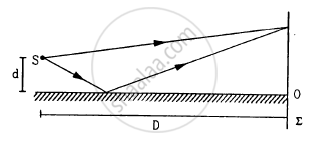Q 22 | Page 382

A long narrow horizontal slit is paced 1 mm above a horizontal plane mirror. The interference between the light coming directly from the slit and that after reflection is seen on a screen 1.0 m away from the slit. Find the fringe-width if the light used has a wavelength of 700 nm.

Q 23 | Page 382

A long narrow horizontal slit is paced 1 mm above a horizontal plane mirror. The interference between the light coming directly from the slit and that after reflection is seen on a screen 1.0 m away from the slit. If the mirror reflects only 64% of the light energy falling on it, what will be the ratio of the maximum to the minimum intensity in the interference pattern observed on the screen?

Q 24 | Page 382

A double slit S1 − S2 is illuminated by a coherent light of wavelength $\lambda.$ The slits are separated by a distance d. A plane mirror is placed in front of the double slit at a distance D1 from it and a screen ∑ is placed behind the double slit at a distance D2 from it (see the following figure). The screen ∑ receives only the light reflected by the mirror. Find the fringe-width of the interference pattern on the screen.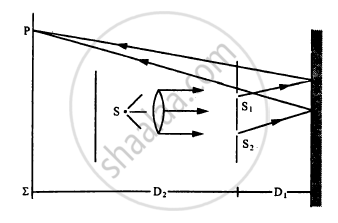Q 25 | Page 382

White coherent light (400 nm-700 nm) is sent through the slits of a Young's double slit experiment (see the following figure). The separation between the slits is 0⋅5 mm and the screen is 50 cm away from the slits. There is a hole in the screen at a point 1⋅0 mm away (along the width of the fringes) from the central line. (a) Which wavelength(s) will be absent in the light coming from the hole? (b) Which wavelength(s) will have a strong intensity?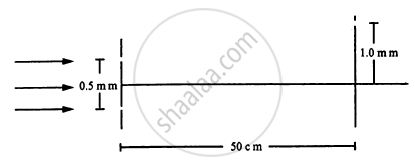Q 26 | Page 382

Consider the arrangement shown in the figure. The distance D is large compared to the separation d between the slits. (a) Find the minimum value of d so that there is a dark fringe at O. (b) Suppose d has this value. Find the distance x at which the next bright fringe is formed. (c) Find the fringe-width.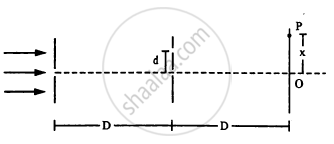Q 27 | Page 382

Two coherent point sources S1 and S2, vibrating in phase, emit light of wavelength $\lambda.$ The separation between the sources is $2\lambda.$ Consider a line passing through S2 and perpendicular to the line S1 S2. What is the smallest distance from S2 where a minimum intensity occurs?

Q 28 | Page 382

The following figure shows three equidistant slits being illuminated by a monochromatic parallel beam of light. Let $B P_0 - A P_0 = \lambda/3\text{ and }D > > \lambda.$ (a) Show that in this case $d = \sqrt{2\lambda D/3}.$ (b) Show that the intensity at P0 is three times the intensity due to any of the three slits individually.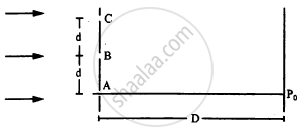Q 29 | Page 382

In a Young's double slit experiment, the separation between the slits = 2.0 mm, the wavelength of the light = 600 nm and the distance of the screen from the slits = 2.0 m. If the intensity at the centre of the central maximum is 0.20 W m−2, what will be the intensity at a point 0.5 cm away from this centre along the width of the fringes?

Q 30 | Page 382

In a Young's double slit interference experiment, the fringe pattern is observed on a screen placed at a distance D from the slits. The slits are separated by a distance d and are illuminated by monochromatic light of wavelength $\lambda.$ Find the distance from the central point where the intensity falls to (a) half the maximum, (b) one-fourth the maximum.

Q 31 | Page 382

In a Young's double slit experiment, $\lambda = 500\text{ nm, d = 1.0 mm and D = 1.0 m.}$ Find the minimum distance from the central maximum for which the intensity is half of the maximum intensity.

Q 32 | Page 382

The line-width of a bright fringe is sometimes defined as the separation between the points on the two sides of the central line where the intensity falls to half the maximum. Find the line-width of a bright fringe in a Young's double slit experiment in terms of $\lambda,$ d and D where the symbols have their usual meanings.

Q 33 | Page 383

Consider the situation shown in the figure. The two slits S1 and S2 placed symmetrically around the central line are illuminated by a monochromatic light of wavelength λ. The separation between the slits is d. The light transmitted by the slits falls on a screen ∑1placed at a distance D from the slits. The slit S3 is at the central line and the slit S4 is at a distance z from S3. Another screen ∑2 is placed a further distance D away from ∑1.Find the ratio of the maximum to minimum intensity observed on ∑2 if z is equal to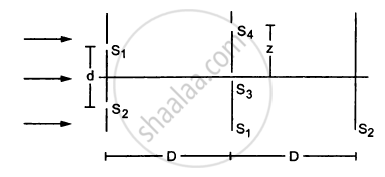(a) $z = \frac{\lambda D}{2d}$

(b) $\frac{\lambda D}{d}$

(c) $\frac{\lambda D}{4d}$

Q 34 | Page 383

Consider the arrangement shown in the figure. By some mechanism, the separation between the slits S3 and S4 can be changed. The intensity is measured at the point P, which is at the common perpendicular bisector of S1S2 and S2S4. When $z = \frac{D\lambda}{2d},$ the intensity measured at P is I. Find the intensity when z is equal to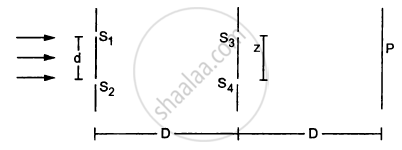(a) $\frac{D\lambda}{d}$

(b) $\frac{3D\lambda}{2d}$  and

(c) $\frac{2D\lambda}{d}$

Q 35 | Page 383

A soap film of thickness 0.0011 mm appears dark when seen by the reflected light of wavelength 580 nm. What is the index of refraction of the soap solution if it is known to be between 1.2 and 1.5?

Q 36 | Page 383

A parallel beam of light of wavelength 560 nm falls on a thin film of oil (refractive index = 1.4). What should be the minimum thickness of the film so that it strongly reflects the light?

Q 37 | Page 383

A parallel beam of white light is incident normally on a water film 1.0 × 10−4 cm thick. Find the wavelengths in the visible range (400 nm − 700 nm) which are strongly transmitted by the film. Refractive index of water = 1.33.

Q 38 | Page 383

A glass surface is coated by an oil film of uniform thickness 1.00 × 10−4 cm. The index of refraction of the oil is 1.25 and that of the glass is 1.50. Find the wavelengths of light in the visible region (400 nm − 750 nm) which are completely transmitted by the oil film under normal incidence.

Q 39 | Page 383

Plane microwaves are incident on a long slit of width 5.0 cm. Calculate the wavelength of the microwaves if the first diffraction minimum is formed at θ = 30°.

Q 40 | Page 383

Light of wavelength 560 nm goes through a pinhole of diameter 0.20 mm and  falls on a wall at a distance of 2.00 m. What will be the radius of the central bright spot formed on the wall?

Q 41 | Page 383

A convex lens of diameter 8.0 cm is used to focus a parallel beam of light of wavelength 620 nm. If the light is focused at a distance of 20 cm from the lens, what would be the radius of the central bright spot formed?

Advertisement Remove all ads

## Chapter 17: Light Waves

Short AnswersMCQOthers## HC Verma solutions for Class 11, 12 Concepts of Physics 1 chapter 17 - Light Waves

HC Verma solutions for Class 11, 12 Concepts of Physics 1 chapter 17 (Light Waves) include all questions with solution and detail explanation. This will clear students doubts about any question and improve application skills while preparing for board exams. The detailed, step-by-step solutions will help you understand the concepts better and clear your confusions, if any. Shaalaa.com has the CBSE Class 11, 12 Concepts of Physics 1 solutions in a manner that help students grasp basic concepts better and faster.

Further, we at Shaalaa.com provide such solutions so that students can prepare for written exams. HC Verma textbook solutions can be a core help for self-study and acts as a perfect self-help guidance for students.

Concepts covered in Class 11, 12 Concepts of Physics 1 chapter 17 Light Waves are Principle of Superposition of Waves, Corpuscular Theory, Speed of Light, Coherent and Incoherent Sources and Sustained Interference of Light, Interference of Light Waves and Young’S Experiment, Reflection and Refraction of Plane Wave at a Plane Surface Using Wave Fronts, Huygens Principle, Introduction of Wave Optics, The Validity of Ray Optics, Seeing the Single Slit Diffraction Pattern, The Single Slit, The Doppler Effect, Reflection of a Plane Wave by a Plane Surface, Refraction at a Rarer Medium, Refraction of a Plane Wave, Refraction of Monochromatic Light, Law of Malus, Width of Central Maximum, Polarisation, Resolving Power of Microscope and Astronomical Telescope, Interference, Proof of Laws of Reflection and Refraction Using Huygen'S Principle, Brewster's Law, Plane Polarised Light, Fraunhofer Diffraction Due to a Single Slit, Coherent and Incoherent Addition of Waves.

Using HC Verma Class 12 solutions Light Waves exercise by students are an easy way to prepare for the exams, as they involve solutions arranged chapter-wise also page wise. The questions involved in HC Verma Solutions are important questions that can be asked in the final exam. Maximum students of CBSE Class 12 prefer HC Verma Textbook Solutions to score more in exam.

Get the free view of chapter 17 Light Waves Class 12 extra questions for Class 11, 12 Concepts of Physics 1 and can use Shaalaa.com to keep it handy for your exam preparation

Advertisement Remove all ads
Share
Notifications

View all notifications

Forgot password?